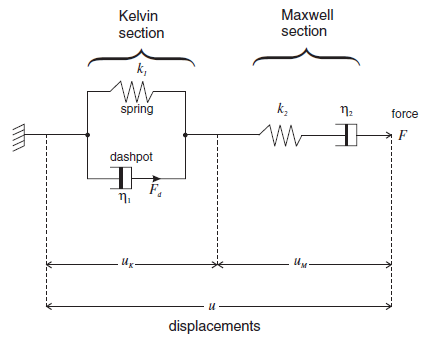FLAC3D Theory and Background • Constitutive Models

# Burgers Model

The Burgers model is composed of a Kelvin model and a Maxwell model connected in series (see Figure 1 for symbol definitions). The equations for the Kelvin sub-model are

(1)$\dot u_k={F_d\over\eta_1}$
(2)$F_d=F-k_1 u_k$

Combining Equations (1) and (2) in finite-difference form,

(3)$u'_k=u_k^\circ+\bigl(\bar F-k_1 \bar u_k\bigr){\Delta t\over\eta_1}$

where $$\bar F$$ and $$\bar u_k$$ correspond to mean values of $$F$$ and $$u_k$$ over the timestep, and the superscripts $${}'$$ and $${}^\circ$$ denote new and old values, respectively. Hence,

(4)$u'_k=u_k^\circ+\bigl\{F'+F^\circ-k_1(u'_k+u_k^\circ)\bigr\} {\Delta t\over 2\eta_1}$

The equation for the Maxwell sub-model is

(5)$\dot u_m={\dot F\over k_2}+{\bar F\over\eta_2}$

which becomes

(6)$u'_m=u_m^\circ+{F'-F^\circ\over k_2}\, +\,\Bigl\{{F'+F^\circ\over2\eta_2}\Bigr\}\Delta t$

when expressed in finite-difference form. Finally, the Kelvin and Maxwell displacement increments combine to give the applied displacement increment,

(7)$u'-u^\circ=u'_m-u_m^\circ\,+\,u'_k-u_k^\circ$

The unknowns in Equations (4), (6), and (7) are $$u'_k$$, $$u'_m$$, and $$F'$$, and the known values are $$u_k^\circ$$ and $$F^\circ$$. The response of the Burgers model is dependent on past history; the state variable that records history information is $$u_k$$, which has an evolution equation derived from Equation (4):

(8)$u'_k={1\over A}\Bigl\{B u_k^\circ+\Bigl(F'+F^\circ\Bigr) {\Delta t\over2\eta_1} \Bigr\}$

where:

(9)$A=1+{k_1\Delta t\over 2\eta_1}$
(10)$B=1-{k_1\Delta t\over 2\eta_1}$

By combining Equations (6) and (7), and substituting $$u'_k$$ from Equation (8), we obtain

(11)$F'={1\over X}\Bigl\{u'-u^\circ+Y F^\circ - \Bigl( {B\over A}-1 \Bigr)u_k^\circ\Bigr\}$

where:

(12)$X={1\over k_2}+{\Delta t\over2\eta_2}+{\Delta t\over2A\eta_1}$
(13)$Y={1\over k_2}-{\Delta t\over2\eta_2}-{\Delta t\over2A\eta_1}$Figure 1: Schematic of the Burgers model with definition of variables.

FISH function for initialization of Kelvin strains

If the stresses are changed in a FLAC3D model with the zone initialize command, the internal Kelvin strains, $$e^K_{ij}$$, will not be compatible with them, and movement will occur until the strains adjust. To avoid this incompatibility, the internal strains may be set to reflect the current values of stresses. The internal Kelvin strains, $$e^K_{ij}$$, are available for user inspection and modification as zone property variables: strain-kelvin-xx, strain-kelvin-yy, etc. An example FISH function to perform this step is given below. This function should be invoked immediately following initialization of stresses by “[SetKStrains(::zone.list)]”.

The following FISH function can be used to set Kelvin strains according to stresses.

SetKStrains.dat

fish operator setKstrains(p_z)
local iflag = 0
if zone.model(p_z) = 'burgers' then
iflag = 1
endif
if zone.model(p_z) = 'burgers-mohr' then
iflag = 1
endif
if iflag = 1 then
local kg2 = 2.0 * zone.prop(p_z, 'shear-kelvin')
if kg2 > 0.0 then
local sig0 = (zone.stress.xx(p_z) + zone.stress.yy(p_z) ...
+ zone.stress.zz(p_z))/3.0
zone.prop(p_z,'strain-kelvin-xx') = ...
(zone.stress.xx(p_z) - sig0) / kg2
zone.prop(p_z,'strain-kelvin-yy') = ...
(zone.stress.yy(p_z) - sig0) / kg2
zone.prop(p_z,'strain-kelvin-zz') = ...
(zone.stress.zz(p_z) - sig0) / kg2
zone.prop(p_z,'strain-kelvin-xy') = ...
zone.stress.xy(p_z) / kg2
zone.prop(p_z,'strain-kelvin-xz') = ...
zone.stress.xz(p_z) / kg2
zone.prop(p_z,'strain-kelvin-yz') = ...
zone.stress.yz(p_z) / kg2
endif
endif
end


burgers Model Properties

Use the following keywords with the zone property (FLAC3D) or block zone property (3DEC) command to set these properties of the Burgers model.

burgers
bulk f

elastic bulk modulus, $$K$$

shear-kelvin f

Kelvin shear modulus, $$G^K$$

shear-maxwell f

Maxwell shear modulus, $$G^M$$

viscosity-kelvin f

Kelvin viscosity, $$n^K$$

viscosity-maxwell f

Maxwell viscosity, $$n^M$$

strain-kelvin-xx f

Kelvin strain, $$e^K_{xx}$$

strain-kelvin-yy f

Kelvin strain, $$e^K_{yy}$$

strain-kelvin-zz f

Kelvin strain, $$e^K_{zz}$$

strain-kelvin-xy f

Kelvin strain, $$e^K_{xy}$$

strain-kelvin-xz f

Kelvin strain, $$e^K_{xz}$$

strain-kelvin-yz f

Kelvin strain, $$e^K_{yz}$$

Notes

• Only one of the two options is required to define the elasticity: bulk modulus $$K$$ and shear modulus $$G$$, or Young’s modulus $$E$$ and Poisson’s ratio $$v$$.
• The creep behavior is triggered by deviatoric stress, while the volumetric behavior does not consider creep.
• If the stresses are changed in a FLAC3D model with the zone initialize command, the internal Kelvin strains, $$e^K_{ij}$$, will not be compatible with them, and movement will occur until the strains adjust. To avoid this incompatibility, the internal strains may be set to reflect the current values of stresses. The internal Kelvin strains, $$e^K_{ij}$$, are available for user inspection and modification. An example FISH function is SetKStrain.f3fis. This function should be invoked immediately following initialization of stresses.